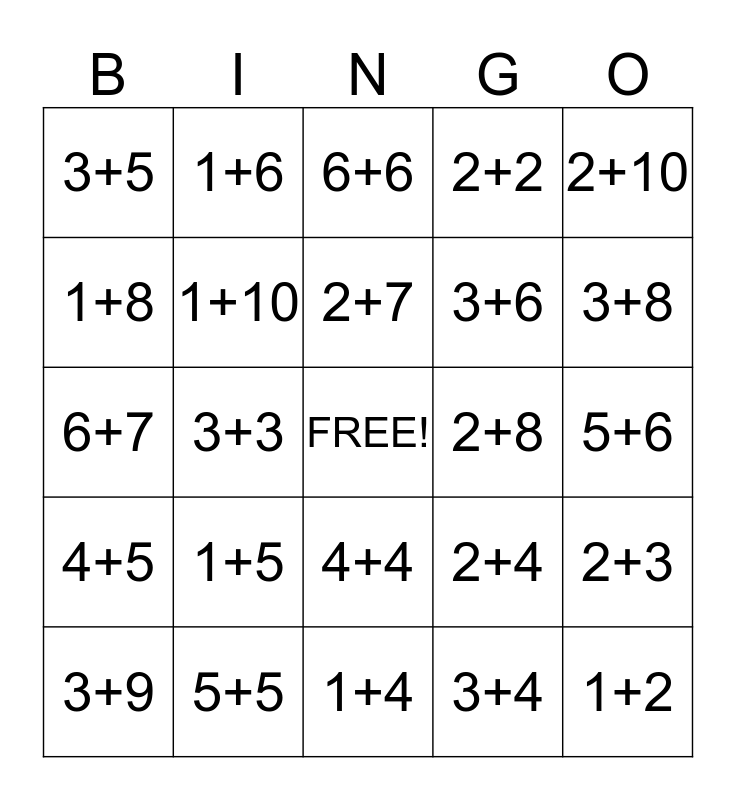This bingo card has a free space and 47 words: 1+0, 1+2, 1+3, 1+4, 1+5, 1+1, 1+6, 1+7, 1+8, 1+9, 1+10, 2+2, 2+3, 2+4, 2+5, 2+6, 2+7, 2+8, 2+9, 2+10, 3+3, 3+4, 3+5, 3+6, 3+7, 3+8, 3+9, 3+10, 4+4, 4+5, 4+6, 4+7, 4+8, 4+9, 4+10, 5+5, 5+6, 5+7, 5+8, 5+9, 5+10, 6+6, 6+7, 6+8, 6+9, 7+7 and 7+8.

## Play Online